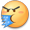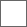### 水中贵族2020-05-26 09:20 广告2020-05-26 09:20 广告{\__/}
( • . •)
/ >⚪️

{\__/}
( • - •)
⚪️< \

{\__/}
( • . •)
/ >⚪️

{\__/}
( • - •)
⚪️< \

{\__/}
( • . •)
/ >⚪️

{\__/}
( • - •)
⚪️< \

Σ(っ °Д °;)っ

{\__/}
( • . •)
/ >⚪️👍⭐️

{\__/}
( • . •)
/>👍

{\__/}
(≖‿≖)✧
👍< \